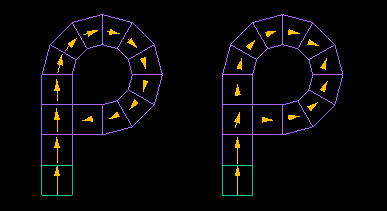## Conventional Magnetic Moment Method

The Magnetic Moment Method divides a body into 3D elements and solves it by assigning variables to the individual magnetic moments of the elements.

＜Calculation Method＞
(1) Suppose there is a coil and several small magnetic bodies.
(2) When an electric current flows through the coil, each magnetic body becomes magnetized.
(3) Each magnetic body generates a magnetic field, which further magnetizes the other magnetic bodies.
(4) Solving the simultaneous equations for this interaction of magnetic bodies determines the magnetization of the magnetic bodies.
(5) Once the magnetization is known, the magnetic field at an arbitrary location can be calculated.Calculation of the magnetic field created by a coil and magnetic material using the " Magnetic Moment Method" (calculation time: less than 1 second).

An electric current is applied to a ring-shaped coil around six magnetic bodies, and the magnetic moment of the magnetic bodies and the spatial magnetic field are calculated.

## Improvements by ELF

However, since the Magnetic Moment Method assumes that the magnetic moment is constant within an element, the magnetic moment does not bend flexibly and becomes highly straight, resulting in cases where it cannot be calculated correctly.

To explain in detail.

Since the polarized magnetic charges cancel each other on the plane between two adjacent elements, the magnetic moments on both sides of the plane are likely to be the same.
If the magnetic moment of an element is constant, then the magnetic moment of a neighboring element is also likely to be the same.

This makes it easier for the magnetic moment to travel in a straight line, which can lead to strange results, such as looping back to a different location. (Looping pattern)On the left is the "Magnetic Moment Method" and on the right is the "ELF's original Integral Element Method" (polarized magnetic charge elements).
The green element at the bottom is the magnet, and the other purple elements are the magnetic elements.

The magnetic flux sent from one magnet element at the bottom goes straight and draws a loop in the Magnetic Moment Method.
In ELF's method, the magnetic flux also branches to the right and solves the problem correctly.

To conventional Surface Charge Method ≫

a:6148 t:2 y:0